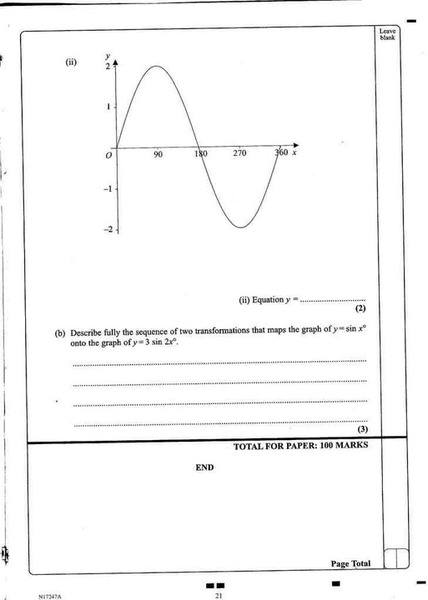# Sin x tranformation

Describe fully the sequence of two transformations that maps the graph y = sinx onto the graph of y = 3sin2x

Well I know that when x = 45 y = 3, when x = 90 y = 0 when x = 135 y = -3 and so on, but tranformations and translation (move), reflection, rotation and englargment. I would presume that the graph is enlarged and then relected, however what is the point of enlargment?

Am I right so far?

ThanksLast edited:

HallsofIvy
Homework Helper
Describe fully the sequence of two transformations that maps the graph y = sinx onto the graph of y = 3sin2x

Well I know that when x = 45 y = 3, when x = 90 y = 0 when x = 135 y = -3 and so on,
Actually, you don't know that- or shouldn't. When sine and cosine are used as functions, rather than to solve functions, the basic definitions require that arguments be interpreted as in radians. (Strictly speaking a mathematician wouldn't think of the argument as an angle at all, but calculators are designed by engineers who do!) What you should know is that when $x= \pi/4$, y= 3, when $x= \pi/2$, y= 0, etc.

but tranformations and translation (move), reflection, rotation and englargment. I would presume that the graph is enlarged and then relected, however what is the point of enlargment?

Am I right so far?

ThanksI'm not sure what you mean by "point of enlargement"- the "enlargement" is uniform along the axes. Your "base function" is sin(x). I prefer to think that anything done after the base function is a change in y, anything done before is a change is x. Here,you multiply x by 2 before taking the sine, then multiply by 3. Okay, multiplying y by 3 stretches (enlarges) the y value (height of the graph) by 3. Multiplying x by 2 before taking sine changes the graph horizontally. Normally, a sine graph goes from 0 to 0 as x changes from 0 to $\pi$. Here, that happens as 2x changes from 0 to $\pi$- in other words as x changes from 0 to [/itex]\pi/2[/itex]. Horizontally, the graph is "shrunk" by 1/2. There is no reflection- that would involve multiplying by -1.part B. How would you answer that? At GCSE, weve never used sin with pi

HallsofIvy
Homework Helper
Yeah, I see that. I don't like but I will try to live with it!

But I told you the transformations are a stretch by a factor of 3 vertically and a "squeeze" by a factor of 1/2 horizontally.

But I told you the transformations are a stretch by a factor of 3 vertically and a "squeeze" by a factor of 1/2 horizontally.

Will that get me the marks in the exam? (it's GCSE)

Thanks

Technically, since it's a sine function you'd need to put that it has an amplitude of 3 instead of 1, and it's period is changed from $$2\pi$$ (or 360 degrees) to $\pi$ (or 180 degrees).

This is for part "b" I mean.

Last edited:
HallsofIvy
Homework Helper
Technically, since it's a sine function you'd need to put that it has an amplitude of 3 instead of 1, and it's period is changed from $$2\pi$$ (or 360 degrees) to $\pi$ (or 180 degrees).

This is for part "b" I mean.

Those statements are true about the graph, but they are not "transformations" and the problem specifically said "describe the sequence of transformations".

As to whether "Will that get me the marks in the exam?" I have no idea! That is how I would answer the question as asked.

Found an interesting document here

http://www.wilsonsschool.sutton.sch.uk/dept/mathematics/stsup/files/page90_6.doc [Broken]

and here:

http://www.bbc.co.uk/schools/gcsebitesize/maths/shapeh/anglesover90rev3.shtml [Broken]

"stretch" is a correct word

Last edited by a moderator: